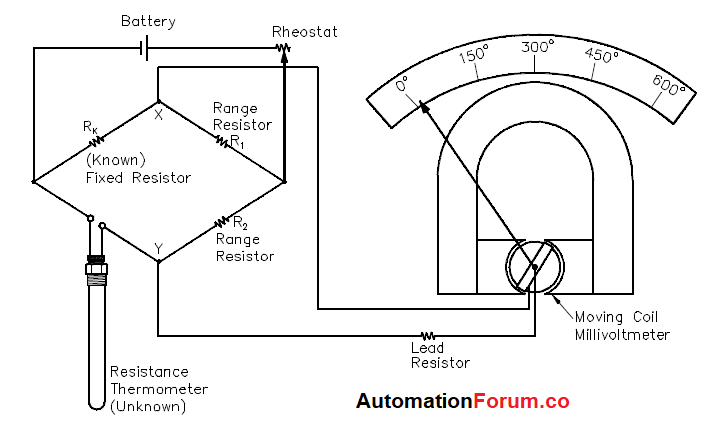# Bridge circuit operation for Temperature measurementA basic bridge circuit which consists of three known resistances, R1, R2, and R3 (variable), an unknown variable resistor RX (RTD), a source of voltage, and a sensitive ammeter.Resistors R1 and R2 are the ratio arms of the bridge. They ratio the two variable resistances for current flow through the ammeter. R3 is a variable resistor known as the standard arm that is adjusted to match the unknown resistor. The sensing ammeter visually displays the current that is flowing through the bridge circuit. Analysis of the circuit shows that when R3 is adjusted so that the ammeter reads zero current, the resistance of both arms of the bridge circuit is the same. Equation below shows the relationship of the resistance between the two arms of the bridge.

R1/R3 = R2/Rx

The value of Rx can be calculated for the bridge during an ammeter zero current condition. Knowing this resistance value provides a baseline point for calibration of the instrument attached to the bridge circuit.

## Bridge circuit operation:

The bridge operates by placing Rx in the circuit, as shown in Figure 8, and then adjusting R3 so that all current flows through the arms of the bridge circuit. When this condition exists, there is no current flow through the ammeter, and the bridge is said to be balanced.

When the bridge is balanced, the currents through each of the arms are exactly proportional. They are equal if R1 = R2. Most of the time the bridge is constructed so that R1 = R2. When this is the case, and the bridge is balanced, then the resistance of Rx is the same as R3, or Rx = R3.

Two types of bridge circuits (unbalanced and balanced) are utilized in resistance thermometer temperature detection circuits:

### Unbalanced bridge circuit:

The unbalanced bridge circuit uses a millivoltmeter that is calibrated in units of temperature that correspond to the RTD resistance.The battery is connected to two opposite points of the bridge circuit. The millivoltmeter is connected to the two remaining points. The rheostat regulates bridge current. The regulated current is divided between the branch with the fixed resistor and range resistor R1, and the branch with the RTD and range resistor R2. As the electrical resistance of the RTD changes, the voltage at points X and Y changes. The millivoltmeter detects the change in voltage caused by unequal division of current in the two branches. The meter can be calibrated in units of temperature because the only changing resistance value is that of the RTD.

### Balanced bridge circuit:

The balanced bridge circuit uses a galvanometer to compare the RTD resistance with that of a fixed resistor. The galvanometer uses a pointer that deflects on either side of zero when the resistance of the arms is not equal. The resistance of the slide wire is adjusted until the galvanometer indicates zero. The value of the slide resistance is then used to determine the temperature of the system being monitored.A slidewire resistor is used to balance the arms of the bridge. The circuit will be in balance whenever the value of the slidewire resistance is such that no current flows through the galvanometer. For each temperature change, there is a new value; therefore, the slider must be moved to a new position to balance the circuit.